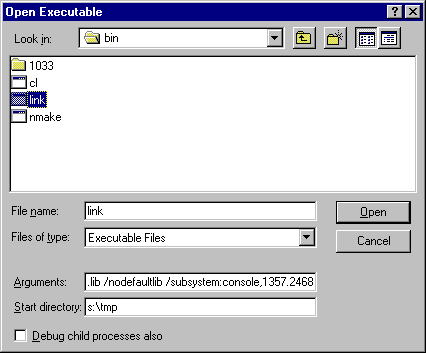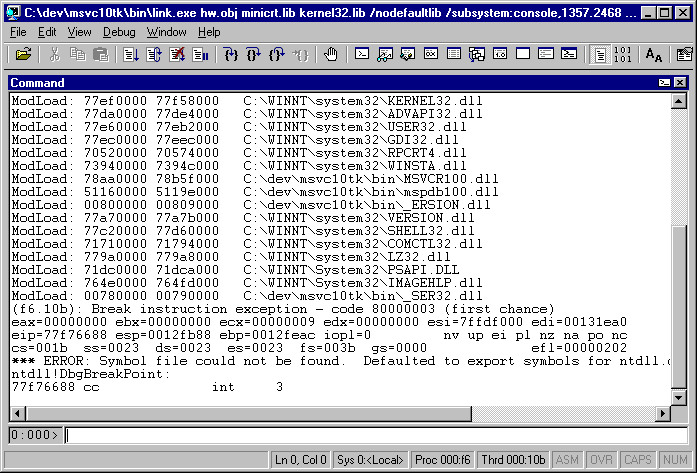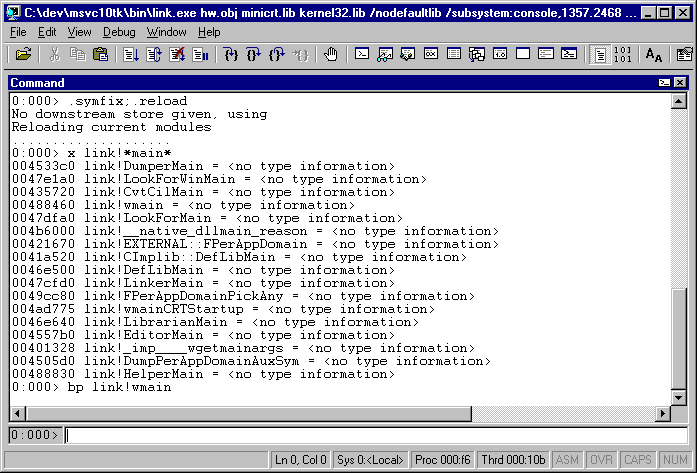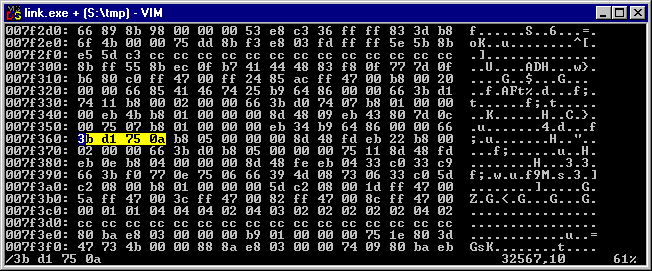# Patching closed software for beginners

25 Feb 2017

In my last entry I showed Visual C++ 2010 running on NT4 and generating an executable than runs on NT4. That last part requires a bit more detail. This version of Visual C++ won't generate programs that work due to the subsystem field, so why did they work for me?

Because it wasn't a stock version of Visual C++ 2010.

In this article we'll walk through an example of how to interpret a closed source program, how to analyze its behavior, and how to ultimately alter that behavior to do what we want. These techniques are well known within many circles, but few tutorials exist to help people get started. The context for this example investigation is the linker's subsystem field generation, but the techniques can be applied to other problems that seem interesting.

Firstly, you'll want to get a serious, machine code level debugger. Many of these exist, although the one I use is WinDbg. I always refer to WinDbg as Microsoft's best kept secret - aside from being a very capable and powerful tool that every serious developer should have, and being freely downloadable, it's surprisingly hard to find. It still has a page on Microsoft's web site which basically tells you to download a WDK or SDK and manually install the component. It has been bundled in these for a long time, so using older WDKs or SDKs may be a good idea, depending on the task at hand.

Because a Windows program is generated by the linker (link.exe), that program is the one that determines what subsystem a given program should have. And, it helpfully includes a switch to specify the subsystem version, although that switch won't let us specify what we want:

```S:\tmp>link hw.obj minicrt.lib kernel32.lib /nodefaultlib /subsystem:console,4.0
Microsoft (R) Incremental Linker Version 10.00.40219.01

LINK : warning LNK4010: invalid subsystem version number 4.0; default subsystem version assumed
```

As mentioned previously, this requirement stems from the libraries and not the linker, and here we're using minicrt, so the restriction makes no sense. The linker is, however, completely happy with totally bogus input:

```S:\tmp>link hw.obj minicrt.lib kernel32.lib /nodefaultlib /subsystem:console,1357.2468
Microsoft (R) Incremental Linker Version 10.00.40219.01
```

This suggests that the linker has a check somewhere for a minimum subsystem version and will fail anything less than that value. So now let's run this again under Windbg and go find that check.Okay, I never said Windbg is user friendly. It's a command line interface, with some UI affordances added, most of which aren't going to help us here since without source code we have nothing to click on.

At this point, WinDbg is waiting at the very top of the program, before anything has happened. It hasn't invoked the program's main() function. Just to help us along a bit, Microsoft publishes limited debugging information about its programs, which means we have enough information to know where the main function is. This means we can load that debug information, and have a breakpoint when we get to main:This shows some commands useful for getting started:

• ".symfix" means "use the default path for debugging symbols"
• ".reload" means "get the debugging symbols"
• "x" means "display addresses of things matching the pattern"
• "bp" means "breakpoint at this location in the code"

Since link was invoked with arguments, the task now is to follow how those arguments are used. There are many ways to do this, but since main() takes arguments as an array, and the program might be using those, let's start by finding that memory.

Here's the first set of simple commands:

• "g" means "resume execution"
• "k" means "stack trace"
• "ub" means "unassemble backwards"
• "dd" means "display 32 bit values"
• "dc" means "display with characters"
```0:000> gh
Breakpoint 0 hit
eax=00c62920 ebx=00000000 ecx=78b5471c edx=00000000 esi=00000001 edi=004c5a2c
eip=00488460 esp=0012ff80 ebp=0012ffc0 iopl=0         nv up ei pl zr na pe nc
cs=001b  ss=0023  ds=0023  es=0023  fs=0038  gs=0000             efl=00000246
00488460 8bff            mov     edi,edi
0:000> k
0012fff0 00000000 KERNEL32!BaseProcessStart+0x40
004ad631 8901            mov     dword ptr [ecx],eax
0:000> dd 004b6630
004b6630  00c62818 00000000 00000000 00000000
0:000> dd 00c62818
00c62818  00c62834 00c6286e 00c6287c 00c62894
00c62828  00c628ae 00c628ca 00000000 003a0043
0:000> dc 00c62834
00c62834  003a0043 0064005c 00760065 006d005c  C.:.\.d.e.v.\.m.
00c62844  00760073 00310063 00740030 005c006b  s.v.c.1.0.t.k.\.
00c62854  00690062 005c006e 0069006c 006b006e  b.i.n.\.l.i.n.k.
00c62864  0065002e 00650078 00680000 002e0077  ..e.x.e...h.w...
00c62874  0062006f 0000006a 0069006d 0069006e  o.b.j...m.i.n.i.
00c62884  00720063 002e0074 0069006c 00000062  c.r.t...l.i.b...
00c62894  0065006b 006e0072 006c0065 00320033  k.e.r.n.e.l.3.2.
00c628a4  006c002e 00620069 002f0000 006f006e  ..l.i.b.../.n.o.
0:000> dc
00c628b4  00650064 00610066 006c0075 006c0074  d.e.f.a.u.l.t.l.
00c628c4  00620069 002f0000 00750073 00730062  i.b.../.s.u.b.s.
00c628d4  00730079 00650074 003a006d 006f0063  y.s.t.e.m.:.c.o.
00c628e4  0073006e 006c006f 002c0065 00330031  n.s.o.l.e.,.1.3.
00c628f4  00370035 0032002e 00360034 00000038  5.7...2.4.6.8...
```

This snippet shows unassembling the instructions before calling the wmain() function. In Microsoft-land, wmain() takes three arguments: argc, argv, and envp. Here we see three arguments being pushed onto the stack, then calling wmain. The second parameter, argv, contains an array of pointers to different arguments in the program's parameters. Presumably for ease of parsing these are all laid out right next to each other, so we basically see the whole parameter string in order with string pointers referring to components. The reason for seeing alternating character and "00" is because these are UTF16 strings, so two bytes per character.

For those new to x86 assembly, it's very well documented online and the great thing about it is how few instructions really exist. Most instructions group into families that you'll see again and again. This makes it tedious to write directly, but it's not hard to follow.

Now we can watch how the memory is accessed. This facility is implemented by the CPU itself, meaning that if you're following along on most software virtual machines, you're out of luck here. Hardware virtualization will implement this correctly.

• "ba" means "break on memory access"
• "u" means "unassemble forwards"
```0:000> ba r 2 00c628e4+c
0:000> gh
Breakpoint 1 hit
eax=00c628f0 ebx=00000000 ecx=ffff0031 edx=00c628cc esi=00c628ca edi=00000002
eip=00498993 esp=0012f2dc ebp=0012f2e8 iopl=0         nv up ei pl nz na po nc
cs=001b  ss=0023  ds=0023  es=0023  fs=0038  gs=0000             efl=00000202
0:000> ub
0049897a 8d4dfc          lea     ecx,[ebp-4]
0049897d 51              push    ecx
0049897e b840654b00      mov     eax,offset link!g_cbExeMapView+0x30 (004b6540)
00498988 8bc6            mov     eax,esi
0049898a 8d5002          lea     edx,[eax+2]
0049898d 8d4900          lea     ecx,[ecx]
00498990 668b08          mov     cx,word ptr [eax]
0:000> u
00498996 6685c9          test    cx,cx
0049899b 2bc2            sub     eax,edx
0049899d d1f8            sar     eax,1
0049899f 8d3c00          lea     edi,[eax+eax]
004989a2 56              push    esi
004989a3 b840654b00      mov     eax,offset link!g_cbExeMapView+0x30 (004b6540)
```

So here we have a piece of code that is reading the memory of interest (the instruction at 498990), moving forward to the next character in memory (498993), if the memory is not a NULL terminator, loop back (498996, 498999.) When it is a NULL terminator, calculate the number of bytes between start and end (49899b), then convert that into characters (49899d), then convert that back into bytes and store it in edi (49899f). Compiler inefficiencies notwithstanding, this code doesn't look like it's what we're after; it's just counting the length. So onwards we go.

```0:000> gh
Breakpoint 1 hit
eax=00c62902 ebx=017c55f0 ecx=00000004 edx=00000000 esi=00c628f2 edi=017c5618
eip=78aa1ed7 esp=0012f2ac ebp=0012f2b4 iopl=0         nv up ei pl nz na pe nc
cs=001b  ss=0023  ds=0023  es=0023  fs=0038  gs=0000             efl=00010206
MSVCR100!memcpy+0x57:
78aa1ed7 f3a5            rep movs dword ptr es:[edi],dword ptr [esi]
0:000> ba r 2 017c5618
```

Another hit - this time in memcpy. So now two pieces of memory have the state we're trying to watch. No problem - we can have multiple breakpoints, so watch the target memory, and keep going.

At this point after hitting 'g' a few times, the code alternates between running those strlen instructions and various memcpy operations. I'm ommitting those for clarity. Then after a few more memcpy's, we have to stop, because we run out of hardware assisted breakpoints. At this point I threw the first memcpy overboard to make way for the most recent one; not ideal, but just an educated guess about which copy is more likely to be of interest.

• "bl" means "list active breakpoints"
• "bc" means "clear specified breakpoint"
```0:000> gh
Breakpoint 1 hit
eax=00c62904 ebx=00000038 ecx=00000004 edx=00000000 esi=00c628f4 edi=017ca210
eip=78aa1ed7 esp=0012d27c ebp=0012d284 iopl=0         nv up ei pl nz na pe nc
cs=001b  ss=0023  ds=0023  es=0023  fs=0038  gs=0000             efl=00010206
MSVCR100!memcpy+0x57:
78aa1ed7 f3a5            rep movs dword ptr es:[edi],dword ptr [esi]
0:000> bl
0 e 00488460     0001 (0001)  0:**** link!wmain
1 e 00c628f0 r 2 0001 (0001)  0:****
2 e 017c5618 r 2 0001 (0001)  0:****
3 e 017c6630 r 2 0001 (0001)  0:****
4 e 017ca1bc r 2 0001 (0001)  0:****
0:000> ba r 2 @edi
0:000> gh
Too many data breakpoints for thread 0
bp5 at 017ca210 failed
WaitForEvent failed
eax=00c62904 ebx=00000038 ecx=00000004 edx=00000000 esi=00c628f4 edi=017ca210
eip=78aa1ed7 esp=0012d27c ebp=0012d284 iopl=0         nv up ei pl nz na pe nc
cs=001b  ss=0023  ds=0023  es=0023  fs=0038  gs=0000             efl=00010206
MSVCR100!memcpy+0x57:
78aa1ed7 f3a5            rep movs dword ptr es:[edi],dword ptr [esi]
0:000> bc 2
0:000> bl
0 e 00488460     0001 (0001)  0:**** link!wmain
1 e 00c628f0 r 2 0001 (0001)  0:****
3 e 017c6630 r 2 0001 (0001)  0:****
4 e 017ca1bc r 2 0001 (0001)  0:****
5 e 017ca210 r 2 0001 (0001)  0:****
0:000> gh
```

And then, after a few hits of wcschr, wcslen and other things that aren't interesting to this task, we hit this curious piece of code. This piece needs to be read a little more carefully:

```Breakpoint 4 hit
eax=00000031 ebx=78b545d0 ecx=017ca1bc edx=00000003 esi=0012d248 edi=00000075
eip=78ab2231 esp=0012ceb0 ebp=0012cec0 iopl=0         nv up ei pl nz ac po cy
cs=001b  ss=0023  ds=0023  es=0023  fs=0038  gs=0000             efl=00000213
MSVCR100!_fgetwc_nolock+0x198:
0:000> ub @eip
MSVCR100!_fgetwc_nolock+0xb:
78ab2212 f6460c40        test    byte ptr [esi+0Ch],40h
78ab2216 57              push    edi
78ab2217 bbd045b578      mov     ebx,offset MSVCR100!__badioinfo (78b545d0)
78ab221c 0f84daa80000    je      MSVCR100!_fgetwc_nolock+0x1b (78abcafc)
78ab2222 834604fe        add     dword ptr [esi+4],0FFFFFFFEh
78ab2226 0f8863200000    js      MSVCR100!_fgetwc_nolock+0x19f (78ab428f)
78ab222c 8b0e            mov     ecx,dword ptr [esi]
78ab222e 0fb701          movzx   eax,word ptr [ecx]
0:000> u
MSVCR100!_fgetwc_nolock+0x198:
78ab2234 890e            mov     dword ptr [esi],ecx
78ab2236 5f              pop     edi
78ab2237 5e              pop     esi
78ab2238 5b              pop     ebx
78ab2239 c9              leave
78ab223a c3              ret
```

This is loading the memory of interest from the memory specified by ecx into the eax register (78ab222e), moving to the next character (78ab2231), and remembering its position (78ab2234). But note what happens next - eax still has the character in it, and the function returns. I misread this code on my first attempt and assumed it was another strlen type function, but it's actually one of the most important parts. So let's move forward a little and see what happens next.

• "t" means "execute one instruction"
• "p" means "execute one function call"
```0:000> t
eax=00000031 ebx=78b545d0 ecx=017ca1be edx=00000003 esi=0012d248 edi=00000075
eip=78ab2234 esp=0012ceb0 ebp=0012cec0 iopl=0         nv up ei pl nz na pe nc
cs=001b  ss=0023  ds=0023  es=0023  fs=0038  gs=0000             efl=00000206
MSVCR100!_fgetwc_nolock+0x19b:
78ab2234 890e            mov     dword ptr [esi],ecx  ds:0023:0012d248=017ca1bc
0:000> t
eax=00000031 ebx=78b545d0 ecx=017ca1be edx=00000003 esi=0012d248 edi=00000075
eip=78ab2236 esp=0012ceb0 ebp=0012cec0 iopl=0         nv up ei pl nz na pe nc
cs=001b  ss=0023  ds=0023  es=0023  fs=0038  gs=0000             efl=00000206
MSVCR100!_fgetwc_nolock+0x1a6:
78ab2236 5f              pop     edi
0:000> t
eax=00000031 ebx=78b545d0 ecx=017ca1be edx=00000003 esi=0012d248 edi=00000075
eip=78ab2237 esp=0012ceb4 ebp=0012cec0 iopl=0         nv up ei pl nz na pe nc
cs=001b  ss=0023  ds=0023  es=0023  fs=0038  gs=0000             efl=00000206
MSVCR100!_fgetwc_nolock+0x1a7:
78ab2237 5e              pop     esi
0:000> t
eax=00000031 ebx=78b545d0 ecx=017ca1be edx=00000003 esi=0012cf68 edi=00000075
eip=78ab2238 esp=0012ceb8 ebp=0012cec0 iopl=0         nv up ei pl nz na pe nc
cs=001b  ss=0023  ds=0023  es=0023  fs=0038  gs=0000             efl=00000206
MSVCR100!_fgetwc_nolock+0x1a8:
78ab2238 5b              pop     ebx
0:000> t
eax=00000031 ebx=0012d2e4 ecx=017ca1be edx=00000003 esi=0012cf68 edi=00000075
eip=78ab2239 esp=0012cebc ebp=0012cec0 iopl=0         nv up ei pl nz na pe nc
cs=001b  ss=0023  ds=0023  es=0023  fs=0038  gs=0000             efl=00000206
MSVCR100!_fgetwc_nolock+0x1a9:
78ab2239 c9              leave
0:000> t
eax=00000031 ebx=0012d2e4 ecx=017ca1be edx=00000003 esi=0012cf68 edi=00000075
eip=78ab223a esp=0012cec4 ebp=0012ced0 iopl=0         nv up ei pl nz na pe nc
cs=001b  ss=0023  ds=0023  es=0023  fs=0038  gs=0000             efl=00000206
MSVCR100!_fgetwc_nolock+0x1aa:
78ab223a c3              ret
0:000> t
eax=00000031 ebx=0012d2e4 ecx=017ca1be edx=00000003 esi=0012cf68 edi=00000075
eip=78ab3d79 esp=0012cec8 ebp=0012ced0 iopl=0         nv up ei pl nz na pe nc
cs=001b  ss=0023  ds=0023  es=0023  fs=0038  gs=0000             efl=00000206
MSVCR100!_output_s_l+0xc70:
78ab3d79 0fb7f8          movzx   edi,ax
```

This code shows returning from a function (the ret instruction at 78ab223a), and the very next instruction is copying the value of the ax register, which contains the character in the arguments string. Clearly the intention was to use that return value in ax. From this point I did a lot of stepping and tracing, which is cut here for brevity. Eventually though the code shows where it wants to save this value, and this seems more interesting than the previous memcpy's, so it's worth following.

```eax=00000031 ebx=0012d2e4 ecx=0000ffff edx=00000003 esi=0012cf68 edi=00000075
eip=78ab3fd1 esp=0012cedc ebp=0012d230 iopl=0         nv up ei pl zr na pe nc
cs=001b  ss=0023  ds=0023  es=0023  fs=0038  gs=0000             efl=00000246
MSVCR100!_winput_s_l+0x342:
78ab3fd1 898530fdffff    mov     dword ptr [ebp-2D0h],eax ss:0023:0012cf60=00000000
0:000> bl
0 e 00488460     0001 (0001)  0:**** link!wmain
1 e 00c628f0 r 2 0001 (0001)  0:****
2 e 0012cec4 r 4 0001 (0001)  0:****
4 e 017ca1bc r 2 0001 (0001)  0:****
5 e 017ca210 r 2 0001 (0001)  0:****
0:000> bc 2
0:000> ba r 2 @ebp-2d0
```

This is still just the first character though, so the code loops around, grabbing more characters. The intention is clearly to convert all of these characters into numbers. There's a core piece of code responsible for doing this conversion. I'm including all of the registers with each step here to make clear what it's really doing:

```eax=00000004 ebx=00000033 ecx=00000033 edx=00000003 esi=00406036 edi=00000075
eip=78ab40a6 esp=0012cedc ebp=0012d230 iopl=0         nv up ei pl nz ac pe nc
cs=001b  ss=0023  ds=0023  es=0023  fs=0038  gs=0000             efl=00000216
MSVCR100!_winput_s_l+0xf32:
78ab40a6 8b850cfdffff    mov     eax,dword ptr [ebp-2F4h] ss:0023:0012cf3c=00000001
0:000>
eax=00000001 ebx=00000033 ecx=00000033 edx=00000003 esi=00406036 edi=00000075
eip=78ab40ac esp=0012cedc ebp=0012d230 iopl=0         nv up ei pl nz ac pe nc
cs=001b  ss=0023  ds=0023  es=0023  fs=0038  gs=0000             efl=00000216
MSVCR100!_winput_s_l+0xf38:
78ab40ac 6bc00a          imul    eax,eax,0Ah
0:000>
eax=0000000a ebx=00000033 ecx=00000033 edx=00000003 esi=00406036 edi=00000075
eip=78ab40af esp=0012cedc ebp=0012d230 iopl=0         nv up ei pl nz na pe nc
cs=001b  ss=0023  ds=0023  es=0023  fs=0038  gs=0000             efl=00000206
MSVCR100!_winput_s_l+0xf70:
78ab40af ff8514fdffff    inc     dword ptr [ebp-2ECh] ss:0023:0012cf44=00000001
0:000>
eax=0000000a ebx=00000033 ecx=00000033 edx=00000003 esi=00406036 edi=00000075
eip=78ab40b5 esp=0012cedc ebp=0012d230 iopl=0         nv up ei pl nz na po nc
cs=001b  ss=0023  ds=0023  es=0023  fs=0038  gs=0000             efl=00000202
MSVCR100!_winput_s_l+0xf76:
78ab40b5 83bdf4fcffff00  cmp     dword ptr [ebp-30Ch],0 ss:0023:0012cf24=00000000
0:000>
eax=0000000a ebx=00000033 ecx=00000033 edx=00000003 esi=00406036 edi=00000075
eip=78ab40bc esp=0012cedc ebp=0012d230 iopl=0         nv up ei pl zr na pe nc
cs=001b  ss=0023  ds=0023  es=0023  fs=0038  gs=0000             efl=00000246
MSVCR100!_winput_s_l+0xf7d:
78ab40bc 0fb7cb          movzx   ecx,bx
0:000>
eax=0000000a ebx=00000033 ecx=00000033 edx=00000003 esi=00406036 edi=00000075
eip=78ab40bf esp=0012cedc ebp=0012d230 iopl=0         nv up ei pl zr na pe nc
cs=001b  ss=0023  ds=0023  es=0023  fs=0038  gs=0000             efl=00000246
MSVCR100!_winput_s_l+0xf80:
78ab40bf 8d4408d0        lea     eax,[eax+ecx-30h]
0:000>
eax=0000000d ebx=00000033 ecx=00000033 edx=00000003 esi=00406036 edi=00000075
eip=78ab40c3 esp=0012cedc ebp=0012d230 iopl=0         nv up ei pl zr na pe nc
cs=001b  ss=0023  ds=0023  es=0023  fs=0038  gs=0000             efl=00000246
MSVCR100!_winput_s_l+0xf84:
78ab40c3 89850cfdffff    mov     dword ptr [ebp-2F4h],eax ss:0023:0012cf3c=00000001
0:000> .formats d
Evaluate expression:
Hex:     0000000d
Decimal: 13
Octal:   00000000015
Binary:  00000000 00000000 00000000 00001101
Chars:   ....
Time:    Wed Dec 31 16:00:13 1969
Float:   low 1.82169e-044 high 0
Double:  6.42285e-323
```

In this sequence, the code is loading the value memory in @ebp-2f4 into eax. At this stage in the loop, that value is 1, because 1 character has already been processed, and that character happened to be 1. Then, that value is multiplied by 0xA (or for humans, multiplied by 10.) The value in ebx, which is the next character, is copied to ecx, and then eax has this value added into it by subtracting 0x30. The character value of '0' is 0x30, so this line is really converting a single character digit into its numerical form and adding it to the previous number. Finally, this number is written back from where it came. And we can see that the value of eax now, which is 0xd, is really the decimal 13. So at this pass of the loop, "1" became "13" because the next digit was added. ".formats" can be used to show this value in a range of formats so we can easily see what the decimal value is.

So execution continues, we do this for all remaining digits, get to the ".", isdigit returns false, the minor subsystem is processed (which we don't have breakpoints for), and then the original numerical form of the major subsystem is reloaded into esi. Now all the pieces fall into place:

```Breakpoint 4 hit
eax=00000001 ebx=78ab086a ecx=3dcc0094 edx=00000003 esi=0000054d edi=017c77a8
eip=0047c04c esp=0012d298 ebp=0012f588 iopl=0         nv up ei pl zr na pe nc
cs=001b  ss=0023  ds=0023  es=0023  fs=0038  gs=0000             efl=00000246
0047c04c 81feffff0000    cmp     esi,0FFFFh
0:000> ub @eip
0047c033 40              inc     eax
0047c034 0056ff          add     byte ptr [esi-1],dl
0047c03f 83f801          cmp     eax,1
0047c048 8b74244c        mov     esi,dword ptr [esp+4Ch]
0:000> u
0047c04c 81feffff0000    cmp     esi,0FFFFh
0047c058 8b5c2444        mov     ebx,dword ptr [esp+44h]
0047c05c 668b5740        mov     dx,word ptr [edi+40h]
0047c060 6a00            push    0
0047c062 53              push    ebx
0047c063 8d4f54          lea     ecx,[edi+54h]
```

Instruction 47c048 loads the value into esi, does sanity checking, and calls FValidSubsystemVersion. Obviously in hindsight this is the function we were looking for in the beginning, and it looks like we've found it. Stepping into this function we eventually see:

```eax=00000200 ebx=000009a4 ecx=00008664 edx=00000000 esi=0000054d edi=017c77a8
eip=0047ff76 esp=0012d288 ebp=0012d288 iopl=0         nv up ei ng nz na pe cy
cs=001b  ss=0023  ds=0023  es=0023  fs=0038  gs=0000             efl=00000287
0047ff76 b805000000      mov     eax,5
0:000>
eax=00000005 ebx=000009a4 ecx=00008664 edx=00000000 esi=0000054d edi=017c77a8
eip=0047ff7b esp=0012d288 ebp=0012d288 iopl=0         nv up ei ng nz na pe cy
cs=001b  ss=0023  ds=0023  es=0023  fs=0038  gs=0000             efl=00000287
0047ff7b 7511            jne     link!FValidSubsystemVersion+0x8e (0047ff8e) [br=1]
0:000>
eax=00000005 ebx=000009a4 ecx=00008664 edx=00000000 esi=0000054d edi=017c77a8
eip=0047ff8e esp=0012d288 ebp=0012d288 iopl=0         nv up ei ng nz na pe cy
cs=001b  ss=0023  ds=0023  es=0023  fs=0038  gs=0000             efl=00000287
0047ff8e 33c9            xor     ecx,ecx
0:000>
eax=00000005 ebx=000009a4 ecx=00000000 edx=00000000 esi=0000054d edi=017c77a8
eip=0047ff90 esp=0012d288 ebp=0012d288 iopl=0         nv up ei pl zr na pe nc
cs=001b  ss=0023  ds=0023  es=0023  fs=0038  gs=0000             efl=00000246
0047ff90 663bf0          cmp     si,ax
0:000> u
0047ff90 663bf0          cmp     si,ax
0047ff97 66394d08        cmp     word ptr [ebp+8],cx
0047ff9d 33c0            xor     eax,eax
0047ff9f 5d              pop     ebp
0047ffa0 c20800          ret     8
0:000> u
0047ffa3 b801000000      mov     eax,1
0047ffa8 5d              pop     ebp
0047ffa9 c20800          ret     8
```

So the value 5 is loaded into eax, and then our silly subsystem which is decimal 1357, or 0x54d in hexadecimal, is compared to it. Looking ahead at the next few instructions, we can see that if the major subsystem specified (si) is above 5, "ja" (jump if above) will execute, taking the system to 47ffa3. If not not equal, which really means it's below 5, the system goes to 47ff9d. If it is equal, 47ff97 executes, which is checking the minor subsystem version. Given that ecx is 0, this code is saying that if the subsystem is >=5.0, the function is returned with eax of 1, but if it's less, this function returns with eax of 0.

We could just change the value 5 at this point, but doing so would mean that this in memory process would be altered only. The real goal is to eliminate this restriction for the next time the linker is executed. So now we need to find the corresponding part of the file and go change that. Unfortunately, running programs are laid out differently in memory to how they're laid out on disk. This is somewhat inconvenient, but not terrible; we can easily search for the contents of this function inside the file. So dumping out this memory, we get the following; note the value of 05 comes from the instruction at 47ff77, at the second line:

```0:000> db 0047ff60
0047ff60  3b d1 75 0a b8 05 00 00-00 8d 48 fd eb 22 b8 00  ;Ñu.¸.....Hýë"¸.
0047ff70  02 00 00 66 3b d0 b8 05-00 00 00 75 11 8d 48 fd  ...f;Ð¸....u..Hý
0047ff80  eb 0e b8 04 00 00 00 8d-48 fe eb 04 33 c0 33 c9  ë.¸.....Hþë.3À3É
0047ff90  66 3b f0 77 0e 75 06 66-39 4d 08 73 06 33 c0 5d  f;ðw.u.f9M.s.3À]
0047ffa0  c2 08 00 b8 01 00 00 00-5d c2 08 00 1d ff 47 00  Â..¸....]Â...ÿG.
```

Switching away from WinDbg and to your favorite hex editor, finding this block is trivial. I used vim + xxd for this:So now we can change the value at 7f377 from '05' to '03' or something more desirable, save the program, and try it out:

```S:\tmp>link hw.obj minicrt.lib kernel32.lib /nodefaultlib /subsystem:console,4.0
Microsoft (R) Incremental Linker Version 10.00.40219.01